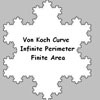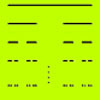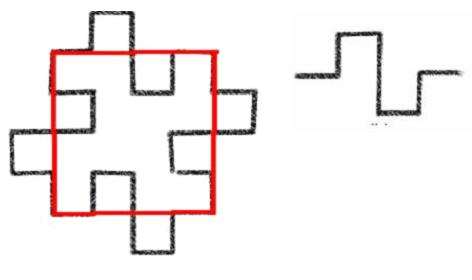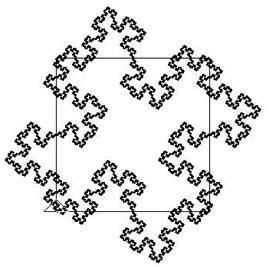#### You may also like### Sierpinski Triangle

What is the total area of the triangles remaining in the nth stage of constructing a Sierpinski Triangle? Work out the dimension of this fractal.### Von Koch Curve

Make a poster using equilateral triangles with sides 27, 9, 3 and 1 units assembled as stage 3 of the Von Koch fractal. Investigate areas & lengths when you repeat a process infinitely often.### How Many Elements Are There in the Cantor Set?

This article gives a proof of the uncountability of the Cantor set.

# Squareflake

##### Age 16 to 18Challenge Level

The diagram below shows Stage $0$ (the red square) and Stage $1$ in the sequence of curves which leads to the squareflake fractal when the process described below is repeated infinitely often. If the original square has side $1$ unit at what stage does the length of the curve begin to exceed $1$ million units? What is the area contained within the fractal curve, what is the length of the curve and what is the dimension of the fractal? What follows is simply information to help you solve this problem.The rule for generating the squareflake fractal curve is to replace each line segment by the zig-zag curve shown above on the right, called the generator. Notice that the generator is made up of $8$ line segments, each one quarter the original length.The curve on the left shows Stage $5$ of the sequence with the Stage $0$ square superimposed but this is not a fractal, it is still early on in the process of forming the fractal. The squareflake fractal contains self similar copies of itself. No matter how much you magnify the fractal curve, zooming in on it, you always see the same structure containing smaller and smaller copies of itself. [If you want to draw fractals for yourself click here to see the Logo program which was used to draw this fractal and for information about downloading a free version of Logo. See First Forward for a twelve part series introducing Logo programming for beginners.]

This problem asks you to work out the dimension of the fractal. To do this you need to use the formula $n=m^d$ where $n$ is the number of copies in the generator of the line segment it replaces, $m$ is the magnification factor, and $d$ is the dimension. (Click here to read how this formula for dimension applies to the dimensions of a line, a square and a cube.)